# 5.6 Electric field lines  (Page 3/5)

 Page 3 / 5

Always keep in mind that field lines serve only as a convenient way to visualize the electric field; they are not physical entities. Although the direction and relative intensity of the electric field can be deduced from a set of field lines, the lines can also be misleading. For example, the field lines drawn to represent the electric field in a region must, by necessity, be discrete. However, the actual electric field in that region exists at every point in space.

Field lines for three groups of discrete charges are shown in [link] . Since the charges in parts (a) and (b) have the same magnitude, the same number of field lines are shown starting from or terminating on each charge. In (c), however, we draw three times as many field lines leaving the $\text{+}3q$ charge as entering the $\text{−}q$ . The field lines that do not terminate at $\text{−}q$ emanate outward from the charge configuration, to infinity.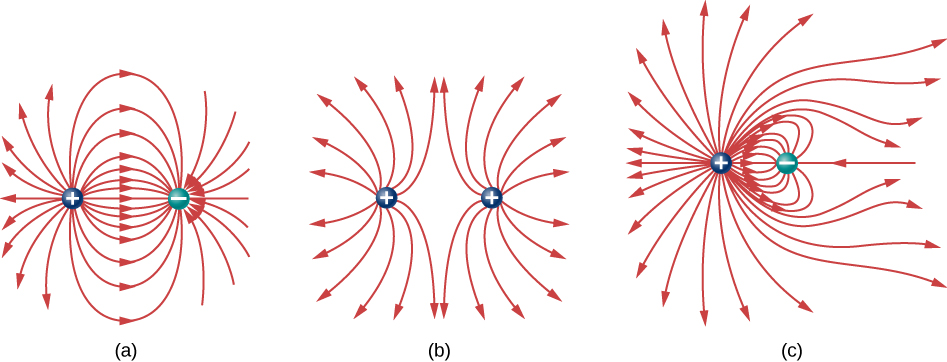Three typical electric field diagrams. (a) A dipole. (b) Two identical charges. (c) Two charges with opposite signs and different magnitudes. Can you tell from the diagram which charge has the larger magnitude?

The ability to construct an accurate electric field diagram is an important, useful skill; it makes it much easier to estimate, predict, and therefore calculate the electric field of a source charge. The best way to develop this skill is with software that allows you to place source charges and then will draw the net field upon request. We strongly urge you to search the Internet for a program. Once you’ve found one you like, run several simulations to get the essential ideas of field diagram construction. Then practice drawing field diagrams, and checking your predictions with the computer-drawn diagrams.

One example of a field-line drawing program is from the PhET “Charges and Fields” simulation.

## Summary

• Electric field diagrams assist in visualizing the field of a source charge.
• The magnitude of the field is proportional to the field line density.
• Field vectors are everywhere tangent to field lines.

## Conceptual questions

If a point charge is released from rest in a uniform electric field, will it follow a field line? Will it do so if the electric field is not uniform?

yes; no

Under what conditions, if any, will the trajectory of a charged particle not follow a field line?

How would you experimentally distinguish an electric field from a gravitational field?

At the surface of Earth, the gravitational field is always directed in toward Earth’s center. An electric field could move a charged particle in a different direction than toward the center of Earth. This would indicate an electric field is present.

A representation of an electric field shows 10 field lines perpendicular to a square plate. How many field lines should pass perpendicularly through the plate to depict a field with twice the magnitude?

What is the ratio of the number of electric field lines leaving a charge 10 q and a charge q ?

10

## Problems

Which of the following electric field lines are incorrect for point charges? Explain why.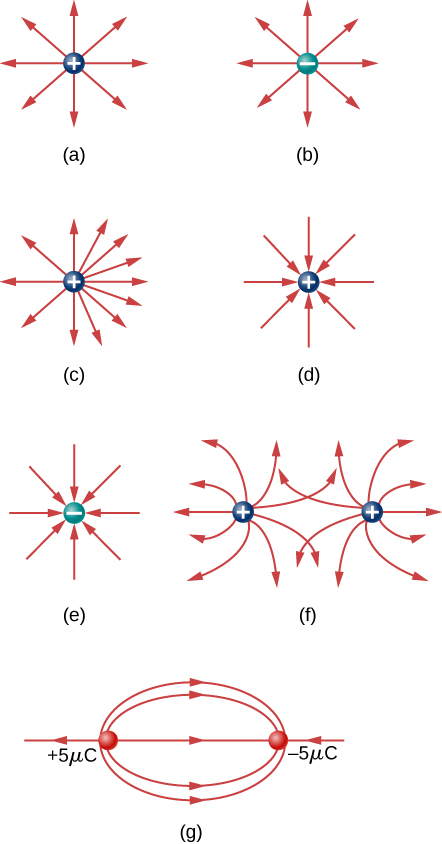In this exercise, you will practice drawing electric field lines. Make sure you represent both the magnitude and direction of the electric field adequately. Note that the number of lines into or out of charges is proportional to the charges.

(a) Draw the electric field lines map for two charges $\text{+}20\phantom{\rule{0.2em}{0ex}}\mu \text{C}$ and $-20\phantom{\rule{0.2em}{0ex}}\mu \text{C}$ situated 5 cm from each other.

(b) Draw the electric field lines map for two charges $\text{+}20\phantom{\rule{0.2em}{0ex}}\mu \text{C}$ and $\text{+}20\phantom{\rule{0.2em}{0ex}}\mu \text{C}$ situated 5 cm from each other.

(c) Draw the electric field lines map for two charges $\text{+}20\phantom{\rule{0.2em}{0ex}}\mu \text{C}$ and $-30\phantom{\rule{0.2em}{0ex}}\mu \text{C}$ situated 5 cm from each other.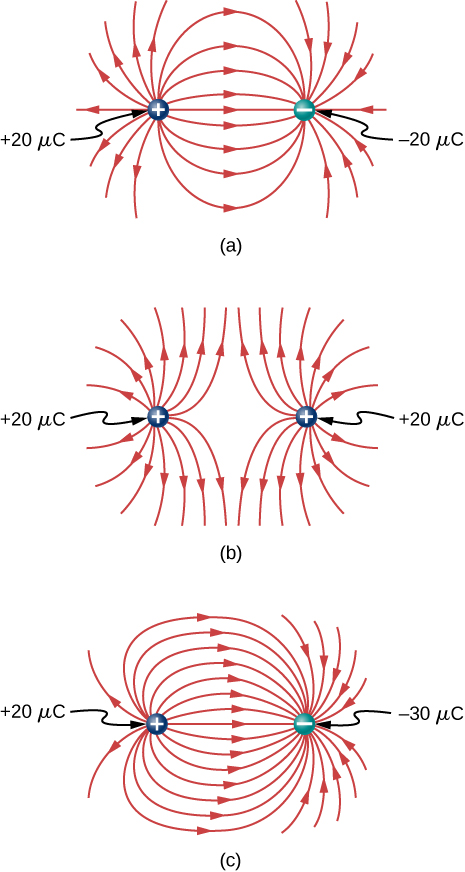Draw the electric field for a system of three particles of charges $\text{+}1\phantom{\rule{0.2em}{0ex}}\mu \text{C},$ $\text{+}2\phantom{\rule{0.2em}{0ex}}\mu \text{C},$ and $-3\phantom{\rule{0.2em}{0ex}}\mu \text{C}$ fixed at the corners of an equilateral triangle of side 2 cm.

Two charges of equal magnitude but opposite sign make up an electric dipole. A quadrupole consists of two electric dipoles are placed anti-parallel at two edges of a square as shown.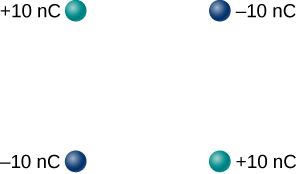Draw the electric field of the charge distribution.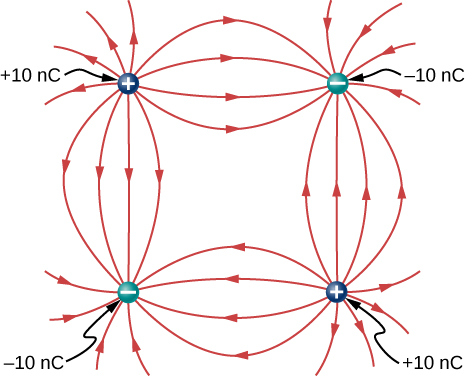Suppose the electric field of an isolated point charge decreased with distance as $1\text{/}{r}^{2+\delta }$ rather than as $1\text{/}{r}^{2}$ . Show that it is then impossible to draw continous field lines so that their number per unit area is proportional to E .

Maxwell's stress tensor is
Yes
doris
neither vector nor scalar
Anil
if 6.0×10^13 electrons are placed on a metal sphere of charge 9.0micro Coulombs, what is the net charge on the sphere
18.51micro Coulombs
ASHOK
Is it possible to find the magnetic field of a circular loop at the centre by using ampere's law?
Is it possible to find the magnetic field of a circular loop at it's centre?
yes
Brother
The density of a gas of relative molecular mass 28 at a certain temperature is 0.90 K kgmcube.The root mean square speed of the gas molecules at that temperature is 602ms.Assuming that the rate of diffusion of a gas in inversely proportional to the square root of its density,calculate the density of
A hot liquid at 80degree Celsius is added to 600g of the same liquid originally at 10 degree Celsius. when the mixture reaches 30 degree Celsius, what will be the total mass of the liquid?
Under which topic
doris
what is electrostatics
Study of charges which are at rest
himanshu
Explain Kinematics
Two equal positive charges are repelling each other. The force on the charge on the left is 3.0 Newtons. Using your notes on Coulomb's law, and the forces acting on each of the charges, what is the force on the charge on the right?
Using the same two positive charges, the left positive charge is increased so that its charge is 4 times LARGER than the charge on the right. Using your notes on Coulomb's law and changes to the charge, once the charge is increased, what is the new force of repulsion between the two positive charges?
Nya
A mass 'm' is attached to a spring oscillates every 5 second. If the mass is increased by a 5 kg, the period increases by 3 second. Find its initial mass 'm'
a hot water tank containing 50,000g of water is heated by an electric immersion heater rated at 3kilowatt,240volt, calculate the current
what is charge
product of current and time
Jaffar
Why always amber gain electrons and fur loose electrons? Why the opposite doesn't happen?
A closely wound search coil has an area of 4cm^2,1000 turns and a resistance of 40ohm. It is connected to a ballistic galvanometer whose resistance is 24 ohm. When coil is rotated from a position parallel to uniform magnetic field to one perpendicular to field,the galvanometer indicates a charge
Using Kirchhoff's rules, when choosing your loops, can you choose a loop that doesn't have a voltage?
how was the check your understand 12.7 solved?By Madison ChristianBy Dan ArielyBy Melinda SalzerBy Hannah ShethBy OpenStaxBy OpenStaxBy Rylee MinllicBy Madison ChristianBy Sarah WarrenBy Brianna Beck